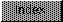```
Taking advantage of other features of the Bullet library is a matter
of setting other engine parameters using one of the SetInfo()
functions with some other level 0 tags.  One interesting feature of
the IntelliFont engine is its ability to rotate glyphs.  By setting
the OT_RotateSin and OT_RotateCos values, the IntelliFont engine can
rotate a glyph's baseline from the glyph origin (the glm_XOrigin and
glm_YOrigin from the GlyphMap structure).

The OT_RotateSin and OT_RotateCos are, respectively, the sine and
cosine of the baseline rotation angle.  The engine can rotate to any
angle.  The sine and cosine must correspond to the same angle and
must be in the sine and cosine value range (0 through 1 inclusive).
The engine does not do any error checking on the sine and cosine
values.  As a result, the engine will yield strange results if the
sine and cosine are from very different angles or if these values are
out of range for sines and cosines (greater than 1).  By default, the
engine sets these values to 0.0 and 1.0, the sine and cosine of 0
degrees.  These values are encoded as fixed point binary fractions
(the negative values are two's complement).

When setting the baseline rotation, an application must set both the
sine and cosine.  It must set OT_RotateSin first, then OT_RotateCos.
An application can set both values in the same SetInfo() function,
but the sine must come first.  For example, to set the rotation angle
to 150 degrees:

The sine of 150 degrees is 0.5 which is 0x00008000 in hex.  The
cosine of 150 degrees is approximately -0.866 which is approximately
0xffff224c in hex (two's complement), so:

.  .  .

if (SetInfo(ge,
OT_RotateSin, 0x8000,
OT_RotateCos, 0xFFFF224C,
TAG_END) == OTERR_Success) /* If SetInfo() returns OTERR_Success, */
/* it worked OK. */
{   /* The baseline rotation has been set, now the application can */
/* render it. */

.  .  .

```